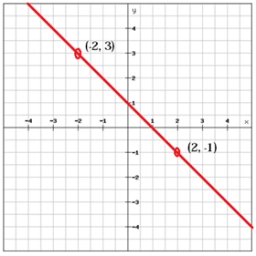# Coefficient 81704

In the equation of the line p: ax-2y+1=0, determine the coefficient a so that the line p:
a) it formed an angle of 120° with the positive direction of the x-axis,
b) passed through point A[3,-2],
c) was parallel to the x-axis,
d) had a direction of k = 4.

a1 =  -3.4641
a2 =  -1.6667
a3 =  0
a4 =  8

### Step-by-step explanation:Did you find an error or inaccuracy? Feel free to write us. Thank you!

Tips for related online calculators
The line slope calculator is helpful for basic calculations in analytic geometry. The coordinates of two points in the plane calculate slope, normal and parametric line equation(s), slope, directional angle, direction vector, the length of the segment, intersections of the coordinate axes, etc.
Do you have a linear equation or system of equations and looking for its solution? Or do you have a quadratic equation?